## What is I (the imaginary unit)?...

Hi,
I wonder what is I in MAPLE?
Obviously I is not an indeterminate

z := a+I*b:
indets(z);

# but

has(z, I)
{a, b}
true


whattype(I);
complex(extended_numeric)


I would have thought that  I was some kind of protected symbol such as Pi, but it's not

attributes(Pi);
attributes(I);
protected


In fact, trying to assign 1 to I just returns an error saying this is not legal assignment

I:=1
Error, illegal use of an object as a name


So, what is I in Maple?

## Quickly finding the lines with errors OR enlargin...

Dear all,

I have a long Maple code.

After running the code, suppose that we get an error in one of the lines.  I can't simply find the mouse cursor in the line which includes the error.

Question 1: How to find quickly which line has the error? What are your solution methods?

Question 2: Enlarging more or highlighting the cursor mark maybe works. Is it possible?

## Error, (in fproc) unable to store 'HFloat...

When i trying to solve the BVP the following error will found

Error, (in fproc) unable to store 'HFloat(1.0141653815612295)+HFloat(0.0020355870386861838)*I' when datatype=float

I have attached my worksheet.

Please anyone help me to get solution to this problem.

Thank you so much

## Explore re-evaluates with variables from end of wo...

Hello Maple friends,

I use Explore() often and noticed something today which seems like either a bug or an error on my usage.

I have a long worksheet where I use Explore at one point to show the behavior of an expression relative to one parameter assigned to a slider. Later in the sheet however, I re-assign some of the variables used in that expression and I call Explore again to see how the expression behaves with the different parameters.

The issue is that when I move the slider on the first explore, the result is incorrect because it seems to be using the values of the variables at the end of the worksheet, not the variables at the point the Explore statement exists.

I would expect Explore to make a copy of the stack at the point it gets called such that the result is correct in the context of its location in the worksheet.

Is this the correct behavior and is there a way to make this work as expected? The only workaround I have in mind is to rename the variable names just to make Explore work, which doesn't seem like a great solution.

Thanks.

## How do i clear this error...

blessing.mw please i really need your help on this, i have been trying to fix this error for a while now.

## on passing keyword argument from one function to a...

I have function that accept keyword argument. From this function, I need to pass this argument to another function using the same signature and using same name for the keyword. But it is not possible to do.

Easier to explain with an example.   This is what I want to do

restart;
foo:=proc(n::integer,{ic::=:=[]})
post_process(n+1,'ic'=ic);
end proc;

post_process:=proc(n::integer,{ic::=:= []})
print("in postprocess, n=",n, "ic=",ic);
end proc;

foo(3,'ic'=(y(0)=5))

Gives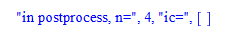The problem is that when calling post_process(), using 'ic'=ic it does not work. Currently I have to change the keyword name as workaround, like this

restart;
foo:=proc(A::integer,{ic::=:=[]})
print("in foo, A=",A, "ic=",ic);
post_process(A,'new_ic'=ic);
end proc;

post_process:=proc(A::integer,{new_ic::=:= []})
print("in postprocess, A=",A, "ic=",new_ic);
end proc;

foo(3,'ic'=(y(0)=5))

Which gives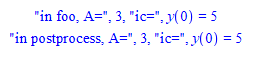Now it passed it. I would have liked to keep same keyword name ic in both function signatures instead of coming up with new name.

Is there a way to do that?

This is not the only problem. The problem with this workaround, is that it does not work when the keyword argument is not passed to the first function. Because now it will use the default values of [].

Hence when calling the post_process() function, it fails due to type mismatch. This now gives an error

restart;
foo:=proc(A::integer,{ic::=:=[]})
print("in foo, A=",A, "ic=",ic);
post_process(A,'new_ic'=ic);
end proc;

post_process:=proc(A::integer,{new_ic::=:= []})
print("in postprocess, A=",A, "ic=",new_ic);
end proc;

foo(3)



Error, (in foo) invalid input: post_process expects value for keyword parameter new_ic to be of type =, but received []

So the way I do this now, is to add an extra check each time, like this

restart;
foo:=proc(A::integer,{ic::=:=[]})
if nargs = 2 then #is ic passed in?
post_process(A,'new_ic'=ic);
else
post_process(A);
fi;
end proc;

post_process:=proc(A::integer,{new_ic::=:= []})
print("in postprocess, A=",A, "ic=",new_ic);
end proc;

foo(3)# now it works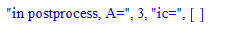But in my actual code, I have more than one keyword argument, and I have to keep checking for correct number of arguments each time, to know which arggument to pass along.

So far, I did not find an easy way around this.

Any suggestion how to do this better? passing keyword argument with default values? from one function to another without getting into these problems?

Maple 2020.2

## Simplify a double sum ...

Dear All

I would like to calculate the five first terms of a  polynomial  sequence

I wrote in maple

sum(x^r*(sum((-1)^s*binomial(r, s)*pochhammer((a+s+1)*d, n), s = 0 .. r))/factorial(r), r = 0 .. n), n = 0 .. 4);

I obtained

0,0,0,0,0

which seems to be false

where is the problem

Best regards

## Why loss of kernel connection?...

This simple set of integrations works correctly and quickly in Maple 17 and other releases, but not in Maple 2020.

psi(x) := (-1)^v*(alpha*GAMMA(k-v)/v!/GAMMA(k-2*v-1)/GAMMA(k-2*v))^(1/2)*exp(-1/2*k*exp(-alpha*x))*                 (k*exp(-alpha*x))^(1/2*k-v-1/2)*LaguerreL(v,k-2*v-1,k/exp(alpha*x))/binomial(k-v-1,v);

Int(eval(%, [k=30.5, v=j, alpha=2.5])^2, x=-1..infinity) =
seq(evalf(Int(eval(%, [k=30.5, v=j, alpha=2.5])^2, x=-1..infinity)), j=0..5);

Every time that I try this simple integration in Maple 2020 I receive a message "kernel connection lost".  Why???

## Bug in Physics Package...

Hi there,

what's wrong with this example:

ph_ex.mw

?

Thank you

## How to find triplets in Lie algebra at which Jacob...

Dear all

I have  Lie commutations for vectors e1, e2, e3, e4, e5, e6 as follow:

[e1, e3] = e3, [e1, e4] = e4, [e1, e5] = e5, [e1, e6] = e6, [e2, e3] = -e5, [e2, e4] = e6, [e3, e5] = e6

for which the command

Query("Jacobi")

returns the false result, which means, the vectors are not closed under Jacobi's identity. How can I find vector triplets for which Jacobi's identity does not hold?

## How do I solve this nonlinear system of Eqs. ?...

Hey everyone!
I have to solve this nonlinear system of equations . For small N (N=8), I tried to do it using solve but it is run forever. I have choosed \gamma to be equal to Pi/4.
test.mw## How to compute Taylor series expansion of sin(x) a...

I tried to expand by the procedures series() and taylor(), but they compute to O(x15).

## plot and smartplot together in one ...

Hi

I have two sets of plots

1. plot([1 - 2*x, -0.6 - 2*x, -1 - 2*x], x = -1 .. 1)

2. smartplot[x, y](map('=', [31*y^2 + 0.19*x^2 + 0.34*x*y - 0.38*y - 0.22*x + 0.07, 0.31*y^2 + 0.19*x^2 + 0.34*x*y - 0.38*y - 0.22*x, 0.31*y^2 + 0.19*x^2 + 0.34*x*y - 0.38*y - 0.22*x + 0.02, 0.31*y^2 + 0.19*x^2 + 0.34*x*y - 0.38*y - 0.22*x + 0.07, 0.31*y^2 + 0.19*x^2 + 0.34*x*y - 0.38*y - 0.22*x + 0.11], 0))

How do i combine the two into one plot?

thanks!

R

## PDEtools:-PolynomialSolutions error when calling p...

Why Maple gives this error and is this a known issue?

restart;
pdsolve( [diff(u(x,t),t)=diff(u(x,t),x)*diff(u(x,t),x$2), u(x,0)=0],u(x,t))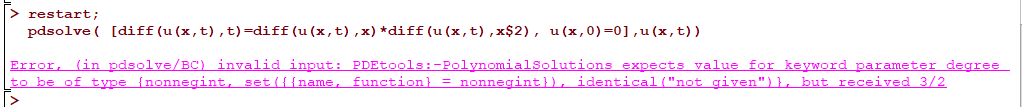Maple 2020.2 with Physics 893 on windows 10. ## The solution of a PDE... Asked by: Dear all, I have a time-fractional PDE as follows. (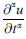denotes Caputo fractional derivative with respect to t)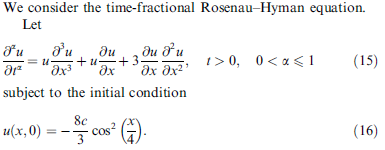for alpha=1, this is a classical PDE and the exact solution is given as follows (in a book)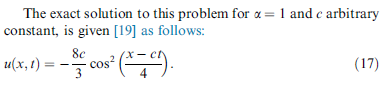Question: 1) for alpha=1, I want to find the L2 errors and L∞ errors in a table. 2) for alpha=0.5, Can Maple find a solution (numeric or exact)? MY TRY: (MAPLE 2020.2) download the code.mw restart: with(plots): PDE:=diff(y(x,t),t)=y(x,t)*diff(y(x,t),x$3)+y(x,t)*diff(y(x,t),x)+3*diff(y(x,t),x)*diff(y(x,t),x\$2) ;

#c is an arbitratry constant
c:=4:
exact_sol:=(x,t)->-8*c/3*(cos ((x-c*t)/4))^2;

# I selected initial and boundary conditions as follows
IBC := { y(x,0)=exact_sol(x,0),y(0,t)=exact_sol(0,t),D(y)(0,t)=D(exact_sol)(0,t),y(1,t)=exact_sol(1,t)};

numeric_sol := pdsolve(PDE,IBC,numeric);

num3d:=numeric_sol:-plot3d(t=0..1,x=0..1,axes=boxed,
color=[0,0,y]);
exact3d:=plot3d(exact_sol(x,t),t=0..1,x=0..1,axes=boxed);
display(exact3d,num3d);

pdetest(y(x,t)=exact_sol(x,t),PDE,IBC);


﻿